# HSSlive: Plus One & Plus Two Notes & Solutions for Kerala State Board

## BSEB Class 10 Maths Chapter 1 Real Numbers Ex 1.2 Textbook Solutions PDF: Download Bihar Board STD 10th Maths Chapter 1 Real Numbers Ex 1.2 Book AnswersBSEB Class 10 Maths Chapter 1 Real Numbers Ex 1.2 Textbook Solutions PDF: Download Bihar Board STD 10th Maths Chapter 1 Real Numbers Ex 1.2 Book Answers

BSEB Class 10th Maths Chapter 1 Real Numbers Ex 1.2 Textbooks Solutions and answers for students are now available in pdf format. Bihar Board Class 10th Maths Chapter 1 Real Numbers Ex 1.2 Book answers and solutions are one of the most important study materials for any student. The Bihar Board Class 10th Maths Chapter 1 Real Numbers Ex 1.2 books are published by the Bihar Board Publishers. These Bihar Board Class 10th Maths Chapter 1 Real Numbers Ex 1.2 textbooks are prepared by a group of expert faculty members. Students can download these BSEB STD 10th Maths Chapter 1 Real Numbers Ex 1.2 book solutions pdf online from this page.

## Bihar Board Class 10th Maths Chapter 1 Real Numbers Ex 1.2 Books Solutions

 Board BSEB Materials Textbook Solutions/Guide Format DOC/PDF Class 10th Subject Maths Chapter 1 Real Numbers Ex 1.2 Chapters All Provider Hsslive

## How to download Bihar Board Class 10th Maths Chapter 1 Real Numbers Ex 1.2 Textbook Solutions Answers PDF Online?

2. Click on the Bihar Board Class 10th Maths Chapter 1 Real Numbers Ex 1.2 Answers.
3. Look for your Bihar Board STD 10th Maths Chapter 1 Real Numbers Ex 1.2 Textbooks PDF.
4. Now download or read the Bihar Board Class 10th Maths Chapter 1 Real Numbers Ex 1.2 Textbook Solutions for PDF Free.

## BSEB Class 10th Maths Chapter 1 Real Numbers Ex 1.2 Textbooks Solutions with Answer PDF Download

Find below the list of all BSEB Class 10th Maths Chapter 1 Real Numbers Ex 1.2 Textbook Solutions for PDF’s for you to download and prepare for the upcoming exams:

## BSEB Bihar Board Class 10th Maths Solutions Chapter 1 Real Numbers Ex 1.2

Question 1.
Express each number as a product of its prime factors:
(i) 140
(ii) 156
(iii) 3825
(iv) 5005
(v) 7429
Solution:
(i) We use the division method as shown below:∴ 140 = 2 × 2 × 5 × 7 = 22 × 5 × 7

(ii) We use the division method as shown below: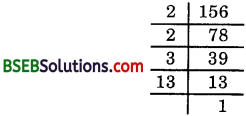∴ 156 = 2 × 2 × 3 × 13 = 22 × 3 × 13

(iii) We use the division method as shown below: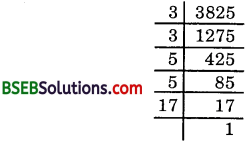∴ 3825 = 3 × 3 × 5 × 5 × 17
= 32 × 52 × 17

(iv) We use the division method as shown below:∴ 5005 = 5 × 7 × 11 × 13

(v) We use the division method as shown below: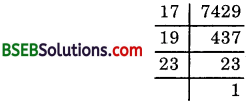∴ 7429 = 17 × 19 × 23

Question 2.
Find the LCM and HCF of the following pairs of integers and verify that LCM × HCF = product of the two numbers.
(i) 26 and 91
(ii) 510 and 92
(iii) 336 and 54
Solution:
(i) 26 and 91∴ LCM of 26 and 91 = 2 × 7 × 13 = 182
and HCF of 26 and 91 = 13
Now, 182 × 13 = 2366 and 26 × 91 = 2366
Thus, 182 × 13 = 26 × 91
Hence verified.

(ii) 510 and 92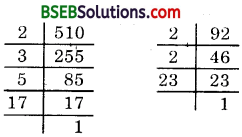510 = 2 × 3 × 5 × 17 and 92 = 2 × 2 × 23
∴ LCM of 510 and 92 = 2 × 2 × 3 × 5 × 17 × 23
= 23460
and HCF of 510 and 92 = 2
Now, 23460 × 2 = 46920 and 510 × 92 = 46920
Thus, 23460 × 2 = 510 × 92
Hence verified.

(iii) 336 and 54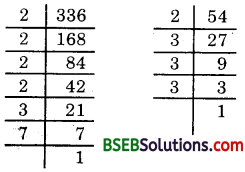336 = 2 × 2 × 2 × 2 × 3 × 7
and 54 = 2 × 3 × 3 × 3
∴ LCM of 336 and 54 = 2 × 2 × 2 × 2 × 3 × 3 × 3 × 7
= 3024
and HCF of 336 and 54 = 2 × 3 = 6
Now, 3024 × 6 = 18144 and 336 × 54 = 18144
Thus, 3024 × 6 = 336 × 54
Hence verified.

Question 3.
Find the LCM and HCF of the following integers by applying the prime factorisation method:
(i) 12, 15 and 21
(ii) 17, 23 and 29
(iii) 8, 9 and 25
Solution:
(i) First we write the prime factorisation of each of the given numbers.
12 = 2 × 2 × 3 = 22 × 3, 15 = 3 × 5 and 21 = 3 × 7
∴ LCM = 22 × 3 × 5 × 7 = 420
and HCF = 3

(ii) First we write the prime factorisation of each of the given numbers.
17 = 1 × 17, 1 × 23 and 1 × 29
[Note that here each of the numbers 17, 23 and 29 is a prime]
∴ LCM = 17 × 23 × 29
= 11339
and HCF = 1
[Note that in 17, 23 and 29, there is no common prime factor]

(iii) First we write the prime factorisation of each of the given numbers.
8 = 2 × 2 × 2 = 23, 9 = 3 × 3 = 32, 25 = 5 × 5 = 52
∴ LCM = 23 × 32 × 52 = 8 × 9 × 25 = 1800
and HCF = 1

Question 4.
Given that IICF (306, 657) = 9, find LCM (306, 657).
Solution:
We know that the product of the HCF and the LCM of two numbers is equal to the product of the given numbers.
∴ HCF (306, 657) × LCM (306, 657) = 306 × 657
So, 9 × LCM (306, 657) = 306 × 657
or LCM (306, 657) = 306×6579
= 22338

Question 5.
Check whether 6n can end with the digit 0 for any natural number n.
Solution:
If the number 6n, for any n, ends with the digit 0, then it is divisible by 5. That is, the prime factorisation of 6n contains the prime 5. This is not possible as the only primes in the factorisation of 6n are 2 and 3 and the uniqueness of the Funtlaniental Theorem of Arithmetic guarantees that there are no other primes in the factorisation of 6n. So, there is no natural number n for which 6n ends with the digit 0.

Question 6.
Explain why 7 × 11 × 13 + 13 and 7 × 6 × 5 × 4 × 3 × 2 × 1 + 5 are composite numbers.
Solution:
Now, 7 × 11 × 13 + 13 = 13 × (7 × 11 × 1 + 1)
= 13 × (77 + 1) = 13 × 78
Hence, it is a composite number.
Again, 7 × 6 × 5 × 4 × 3 × 1 × 1 + 5
= 5 × (7 × 6 × 4 × 3 × 1 × 1 + 1)
So, it is a composite number.

Question 7.
There is a circular path around a sports field. Sonia takes 18 minutes to drive one round of the field, while Ravi takes 12 minutes for the same. Suppose they both start at the same point and at the same time, and go in the same direction. After how many minutes will they again at the starting point?
Solution:
They will be again at the starting point after common multiples of 18 and 12 minutes. To find the LCM of 18 and 12, we have: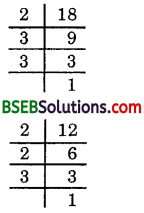LCM of 18 and 12 = 2 × 2 × 3 × 3 = 36
So, Sonia and Ravi will meet again at the starting point after 36 minutes.

## Bihar Board Class 10th Maths Chapter 1 Real Numbers Ex 1.2 Textbooks for Exam Preparations

Bihar Board Class 10th Maths Chapter 1 Real Numbers Ex 1.2 Textbook Solutions can be of great help in your Bihar Board Class 10th Maths Chapter 1 Real Numbers Ex 1.2 exam preparation. The BSEB STD 10th Maths Chapter 1 Real Numbers Ex 1.2 Textbooks study material, used with the English medium textbooks, can help you complete the entire Class 10th Maths Chapter 1 Real Numbers Ex 1.2 Books State Board syllabus with maximum efficiency.

## FAQs Regarding Bihar Board Class 10th Maths Chapter 1 Real Numbers Ex 1.2 Textbook Solutions

#### Can we get a Bihar Board Book PDF for all Classes?

Yes you can get Bihar Board Text Book PDF for all classes using the links provided in the above article.

## Important Terms

Bihar Board Class 10th Maths Chapter 1 Real Numbers Ex 1.2, BSEB Class 10th Maths Chapter 1 Real Numbers Ex 1.2 Textbooks, Bihar Board Class 10th Maths Chapter 1 Real Numbers Ex 1.2, Bihar Board Class 10th Maths Chapter 1 Real Numbers Ex 1.2 Textbook solutions, BSEB Class 10th Maths Chapter 1 Real Numbers Ex 1.2 Textbooks Solutions, Bihar Board STD 10th Maths Chapter 1 Real Numbers Ex 1.2, BSEB STD 10th Maths Chapter 1 Real Numbers Ex 1.2 Textbooks, Bihar Board STD 10th Maths Chapter 1 Real Numbers Ex 1.2, Bihar Board STD 10th Maths Chapter 1 Real Numbers Ex 1.2 Textbook solutions, BSEB STD 10th Maths Chapter 1 Real Numbers Ex 1.2 Textbooks Solutions,
Share: